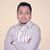## TyroCity is a community of amazing learners

We're a place where students share, stay up-to-date, learn and grow.

# Discussion on: What is the concept of diminishing returns? What role does the Price Line play for the equilibrium condition?According to Brue (1993), "To modern economists, diminishing returns in the most basic sense occurs when marginal product falls as a rising amount of a variable homogeneous input is applied to a fixed input.” As the term "diminish returns” means a reduction on returns, so it applies that at first when the additional input is added then the output also increases at that rate. However, the more and more single input is added then the output tends to increase at decreasing rate (Guell, 2015). It means that at a particular point the output increases but at a slower rate. After that, it starts to diminish the extra output of the group despite the addition of per unit input.

Now, I would like to define the law of diminishing return trough graphical representation.

Figure: Law of Diminishing Return

In the above graph what we can depict is that at point A the production starts as a firm requires workers to yield the output. Then after at point B, additional workers are added to increase the productivity. From point B the outcome slowly starts to increase. Again the input i.e. additional workers are added to increase the productivity which as a result a steeper slope is created. This means that from the point B to A the output has skyrocketed. Since the point C to point D the output has increased but at the decreasing rate despite the addition of workers. This means that from the point D the law of diminishing return prevailed especially holding other factors of production constant.

I would like to illustrate the law of diminishing return trough numerical representation.

Figure: Numerical Illustration of Law of Diminishing Return

In the above table, there consist input (chefs), total output and productivity of extra output of all chefs. As we can see that initially the production commences from zero input and output and as the production takes its course additional input (chefs) i.e. 3 is added which as a consequence yield the output of 10. Likewise, the additional chefs are further added 3 and 5 which as a result yielded 25 and 55 output sequentially. Up to this point (addition of 7 chefs), the production has increased and reached to the optimum point i.e. 55 extra output by all chefs. Furthermore, when the 10 additional input or chefs are added then the production has increased but at a decreasing rate (50 output by the group). Since then each additional chefs or input has increased but at the decreasing rate.

References

Brue, S. L. (1993). Retrospectives: The law of diminishing returns. Journal of Economic Perspectives, 7 (3), 185-192.

Guell, Robert C. (2015). Issues in Economics Today , 7th edition- 2015 ISBN: 978-0078021817